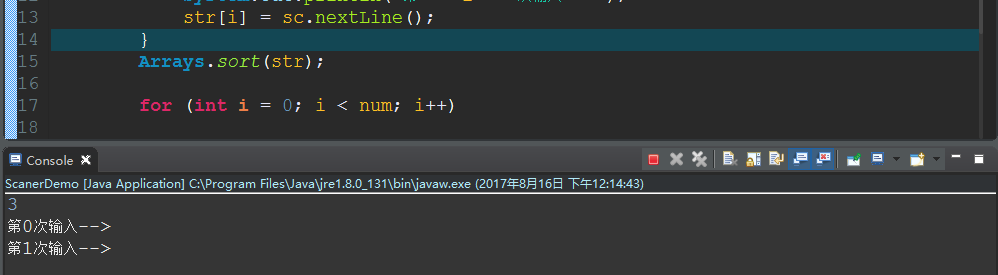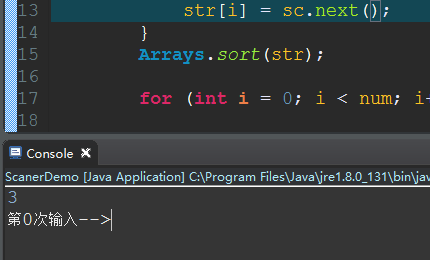package excise2;
import java.util.*;
public class TestExe1 {

`````` public static void main(String args[])
{
Scanner sc=new Scanner(System.in);
int num=sc.nextInt();
String []str=new String[num];

for(int i=0;i<num;i++)
{

str[i]=sc.nextLine();
}

Arrays.sort(str);

for(int i=0;i<num;i++)
{
System.out.println(str[i]);
}
``````

}
}

``````
``````

3个回答int num=sc.nextInt();
String []str=new String[num];

str[i]=sc.nextLine();

，当next()、nextInt()、nextDouble()等等这些之后，你如果不再加一条in.nextLine()的话，下面如果用到了类似str = in.nextLine(); 这条语句的话，会首先读取上面next()、nextInt()、nextDouble()等等这些语句的回车作为一条默认的数据

next()、nextInt()、nextDouble()等等这些 之后，再用一个in.nextLine()这个来截取上面的一个回车操作，后面的nextLine()在第一次才能起作用；

``````  public static void main(String args[])
{
Scanner sc=new Scanner(System.in);
int num=sc.nextInt();
sc.nextLine();
String []str=new String[num];

for(int i=0;i<num;i++)
{
Scanner sb=new Scanner(System.in);
str[i]=sb.nextLine();
}

Arrays.sort(str);

for(int i=0;i<num;i++)
{
System.out.println(str[i]);
}
}

``````